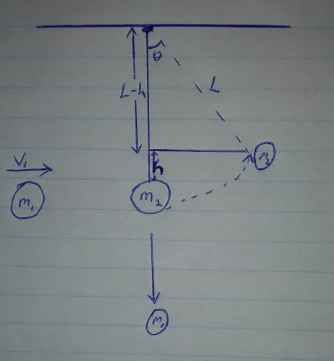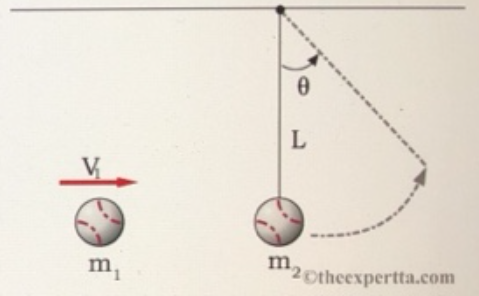# Problem: A baseball of mass m1 = 0.39 kg is thrown at another ball hanging from the ceiling by a length of string L = 1.95 m. The second ball m2 = 0.85 kg is initially at rest while the baseball has an initial horizontal velocity of V1 = 3.5 m/s. After the collision, the first baseball falls straight down (no horizontal velocity.)What is the angle that the string makes with the vertical at the highest point of travel in degrees?

###### FREE Expert Solution

From the law of conservation of momentum:

$\overline{){{\mathbf{m}}}_{{\mathbf{1}}}{{\mathbf{v}}}_{{\mathbf{1}}}{\mathbf{=}}{{\mathbf{m}}}_{{\mathbf{2}}}{{\mathbf{v}}}_{{\mathbf{2}}}}$

Law of conservation of energy;

$\overline{){{\mathbf{K}}}_{{\mathbf{i}}}{\mathbf{+}}{{\mathbf{U}}}_{{\mathbf{i}}}{\mathbf{+}}{\mathbf{w}}{\mathbf{=}}{{\mathbf{K}}}_{{\mathbf{f}}}{\mathbf{+}}{{\mathbf{U}}}_{{\mathbf{f}}}}$

Let's consider the following diagram, after the collision:###### Problem Details

A baseball of mass m1 = 0.39 kg is thrown at another ball hanging from the ceiling by a length of string L = 1.95 m. The second ball m2 = 0.85 kg is initially at rest while the baseball has an initial horizontal velocity of V1 = 3.5 m/s. After the collision, the first baseball falls straight down (no horizontal velocity.)What is the angle that the string makes with the vertical at the highest point of travel in degrees?